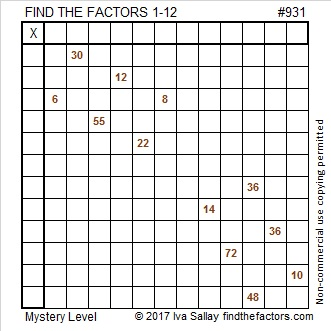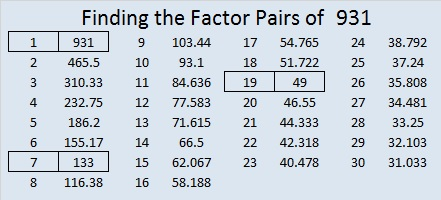# 931 Candy Bar Mystery

What kind of candy bars might these be? That is the mystery. What are your favorite candy bars? My favorites are Snickers, 100 Grand, and Baby Ruth.

What level is this puzzle? I’m not telling. Figuring that out is part of the fun. Give this mystery level puzzle a try. If you can’t solve it, it will be a trick, but if you can, it will be a treat.Print the puzzles or type the solution on this excel file: 12 factors 923-931

931 is the sum of consecutive prime numbers three different ways:
It is the sum of the 15 prime numbers from 31 to 97,
the sum of the 11 prime numbers from 61 to 107, and
these three consecutive primes, 307 + 311 + 313 = 931.

Here’s 931 in a few different bases:
It’s repdigit 777 in BASE 11, because 7(121) + 7(11) + 7(1) = 7(133) = 931, and
repdigit 111 in BASE 30, because 1(900) + 1(30) + 1(1) = 931.
931 is palindrome 3A3 in BASE 16 (A is 10 base 10), because 3(16²) + 10(16) + 3(1) = 931

• 931 is a composite number.
• Prime factorization: 931 = 7 × 7 × 19, which can be written 931 = 7² × 19
• The exponents in the prime factorization are 2 and 1. Adding one to each and multiplying we get (2 + 1)(1 + 1) = 3 × 2  = 6. Therefore 931 has exactly 6 factors.
• Factors of 931: 1, 7, 19, 49, 133, 931
• Factor pairs: 931 = 1 × 931, 7 × 133, or 19 × 49
• Taking the factor pair with the largest square number factor, we get √931 = (√49)(√19) = 7√19 ≈ 30.51229This site uses Akismet to reduce spam. Learn how your comment data is processed.